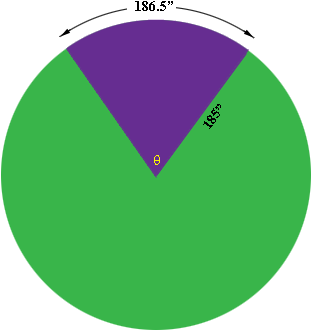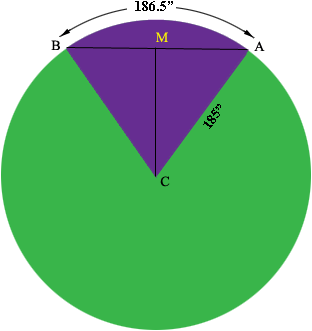SEARCH HOMEMath Central Quandaries & QueriesQuestion from larry, a parent: i have a radius of 185". and an arc length of 186.5". how do i find the arc degrees or cord length?Hi Larry,The circumference of a circle of radius r = 185 inches is 2 π r = 370 π inches. The length of your arc is a fraction of the circumference, in fact it is 186.5/(370 π) = 0.1604 of the circumference. By the symmetry of the circle the angle θ is the same fraction o 360o. Thus

θ = 0.1604 × 360o = 57.76o.

To find the cord length I let M be the midpoint of the cordTriangle AMC is a right triangle and the measure of angle MCA = 57.76o/2 = 28.88o. Thus

sin(28.88o) = |AM|/185"

so

|AB| = 2 × |AM| = 2 × 185" × sin(28.88o)

HarleyMath Central is supported by the University of Regina and The Pacific Institute for the Mathematical Sciences.